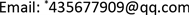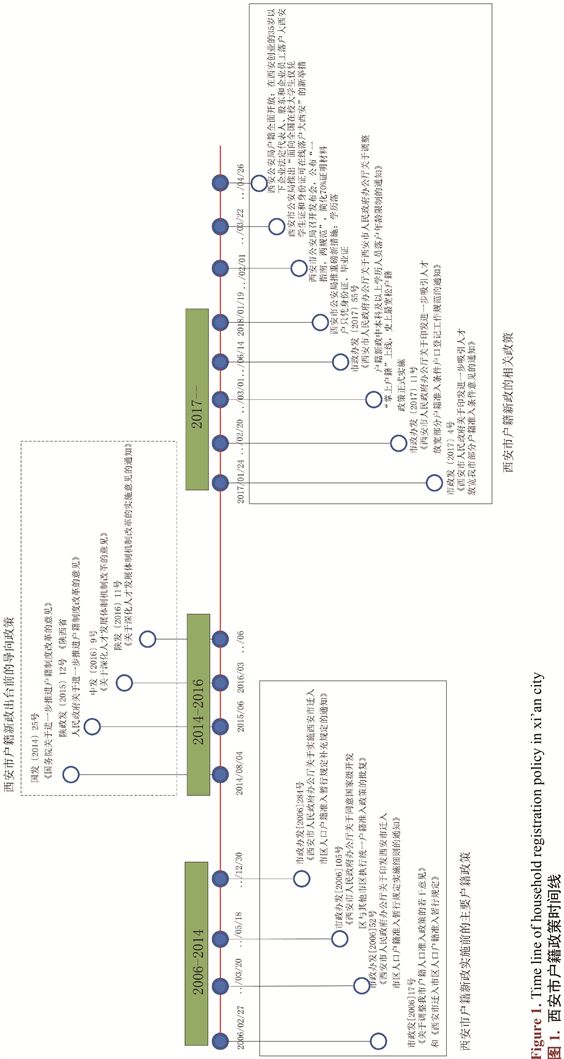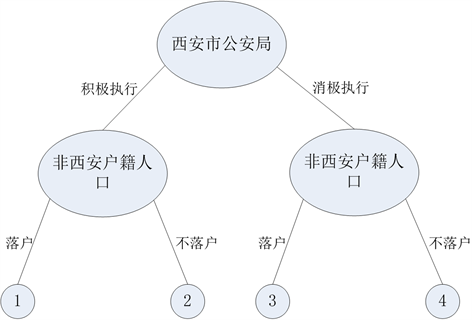﻿ 博弈视角下的公共政策过程分析——以西安市户籍新政为例 Analysis of Public Policy Process from the Perspective of Game—Taking the New Deal of Household Registration in Xi’an as an Example

Advances in Social Sciences
Vol. 08  No. 04 ( 2019 ), Article ID: 29986 , 14 pages
10.12677/ASS.2019.84096

Analysis of Public Policy Process from the Perspective of Game

—Taking the New Deal of Household Registration in Xi’an as an Example

Lichao Meng, Shuang Lai*, Ningjuan Li

College of Humanities and Economics, Northwestern Polytechnical University, Xi’an ShaanxiReceived: Apr. 7th, 2019; accepted: Apr. 21st, 2019; published: Apr. 28th, 2019ABSTRACT

In order to explain the action logic of the participants in the process of public policy, with the help of game theory and taking the new household registration policy in Xi’an as an example, this paper constructs the game model of “Xi’an Municipal Government-other municipal governments” and “Xi’an Public Security Bureau-non-Xi’an household registration population”, describes the formulation and implementation process of the new household registration policy in Xi’an, and explains the essential reasons for the policy subjects to make strategic choices through cost-benefit analysis. The study finds that the fundamental motive force for Xi’an Municipal Government to formulate the new household registration policy is to seek the huge benefits brought by talents for the future development of the city. Under the vigorous promotion of the Xi’an municipal government, the Xi’an Public Security Bureau will undoubtedly gain departmental benefits, higher-level awards and future benefits by actively implementing the new household registration policy; after measuring the existing and future benefits of settled in Xi’an, the migrant population, encouraged by the reward and subsidy policy of Xi’an, will choose to settle in Xi'an for the sake of optimizing the future development of Xi’an.

Keywords:Public Policy Process, Game Theory, New Policy of Xi’an Household Registration

——以西安市户籍新政为例Copyright © 2019 by author(s) and Hans Publishers Inc.

This work is licensed under the Creative Commons Attribution International License (CC BY).

http://creativecommons.org/licenses/by/4.0/1. 引言

2. 相关理论与文献综述

3. 博弈视角下的西安户籍新政

3.1. 西安市户籍新政Table 1. Comparison of permanent resident population between Xi’an and Chengdu from the end of 2010 to the end of 2015Table 2. Previous household registration policies

3.2. 政策过程中的博弈

1) 政策制定中的博弈Figure 2. Game tree of “Xi’an municipal government-other municipal government”Table 5. Game income matrix table of “Xi’an municipal government-other municipal government”Table 6. Definition table of game parameters of “Xi’an municipal government-other municipal government”

$\begin{array}{c}{\text{V}}_{1}\left(制定\right)=\text{p}\ast \text{q}\ast \left(\text{Rx1}+\text{Rx2}+\text{Rx3}+\text{Rx4}-\text{Cx1}-\text{Cx2}\right)\\ \text{\hspace{0.17em}}+\text{p}\ast \left(\text{1}-\text{q}\right)\ast \left(\text{Rx1}+\text{Rx2}+\text{Rx3}+\text{Rx4}-\text{Cx1}-\text{Cx2}\right)\\ =\text{p}\ast \left(\text{Rx1}+\text{Rx2}+\text{Rx3}+\text{Rx4}-\text{Cx1}-\text{Cx2}\right);\end{array}$ 公式1

$\begin{array}{c}{\text{V}}_{1}\left(不制定\right)=\left(\text{1}-\text{p}\right)\ast \text{q}\ast \left(-\left(\text{Cx3}+\text{Wx1}\right)\right)+\left(\text{1}-\text{p}\right)\ast \left(\text{1}-\text{q}\right)\ast \left(-\left(\text{Cx3}+\text{Wx1}\right)\right)\\ =\left(\text{1}-\text{p}\right)\ast \left(-\left(\text{Cx3}+\text{Wx1}\right)\right);\end{array}$ 公式2

2) 政策执行中的博弈Figure 3. Game tree of “Xi’an public security bureau-non-Xi’an resident registration population”Table 7. “Xi’an public security bureau-non-Xi’an resident registration population” game income matrix tableTable 8. Definition table of game parameters of “Xi’an public security bureau-non-xi'an resident registration population”

$\begin{array}{c}{\text{V}}_{\text{1}}\left(积极执行\right)=\text{p}\ast \text{q}\ast \left(\text{Rp1}+\text{Rp2}+\text{Rp3}-\text{Cp1}\right)+\text{p}\ast \left(\text{1}-\text{q}\right)\ast \left(\text{Rp1}+\text{Rp2}+\text{Rp3}-\text{Cp1}\right)\\ =\text{p}*\left(\text{Rp1}+\text{Rp2}+\text{Rp3}-\text{Cp1}\right);\end{array}$ 公式3

$\begin{array}{c}{\text{V}}_{\text{1}}\left(消极执行\right)=\left(\text{1}-\text{p}\right)\ast \text{q}\ast \left(-\left(\text{Cp2}+\text{Wp1}+\text{Wp2}\right)\right)+\left(\text{1}-\text{p}\right)\ast \left(\text{1}-\text{q}\right)\ast \left(-\left(\text{Cp2}+\text{Wp1}+\text{Wp2}\right)\right)\\ =\left(\text{1}-\text{p}\right)\ast \left(-\left(\text{Cp2}+\text{Wp1}+\text{Wp2}\right)\right);\end{array}$ 公式4

$\begin{array}{c}{\text{V}}_{\text{2}}\left(落户\right)=\text{q}\ast \text{p}\ast \left(\text{Rn1}+\text{Rn2}-\text{Cn1}-\text{Cn2}\right)+\text{q}\ast \left(\text{1}-\text{p}\right)\ast \left(\text{Rn1}+\text{Rn2}-\text{Cn1}-\text{Cn2}\right)\\ =\text{q}\ast \left(\text{Rn1}+\text{Rn2}-\text{Cn1}-\text{Cn2}\right);\end{array}$ 公式5

${\text{V}}_{\text{2}}\left(不落户\right)=\left(\text{1}-\text{q}\right)\ast \text{p}\ast \left(-\text{Wn1}\right)+\left(\text{1}-\text{q}\right)\ast \left(\text{1}-\text{p}\right)\ast \left(-\text{Wn1}\right)=\left(\text{1}-\text{q}\right)\ast \left(-\text{Wn1}\right);$ 公式6

3) 政策评估与政策终结的博弈

4. 小结

Analysis of Public Policy Process from the Perspective of Game—Taking the New Deal of Household Registration in Xi’an as an Example[J]. 社会科学前沿, 2019, 08(04): 675-688. https://doi.org/10.12677/ASS.2019.84096

1. 1. Easton, D. (1953) The Political System: An Inquiry into the State of Political Science. A.A. Knopf, New York, 129.

2. 2. 詹姆斯•E•安德森. 公共政策制定(第五版) [M]. 北京: 中国人民大学出版社, 2009: 3.

3. 3. Lasswell, H.D. (1971) A Preview of Policy Sciences. Elsevier, New York.

4. 4. 丹尼尔•W. 经济利益与经济制度: 公共政策的理论基础[M]. 布罗姆利, 陈郁, 译. 上海: 格致出版社, 2012: 5-7.

5. 5. 薛澜, 林泽梁. 公共政策过程的三种视角及其对中国政策研究的启示[J]. 中国行政管理, 2013(5): 41-46.

6. 6. 丁宁宁, 刘曼曼. 我国公共政策执行过程的修正型框架: 基于多元协调的视角[J]. 贵州社会科学, 2014(9): 136-141.

7. 7. 姚刚. 国外公共政策绩效评估研究与借鉴[J]. 深圳大学学报(人文社会科学版), 2008(4): 80-85.

8. 8. 姜国兵. 公共政策绩效评估体系建构初探[J]. 广东行政学院学报, 2012, 24(6): 7-12 + 19.

9. 9. 曲纵翔. 公共政策终结: 基于“过程”角度的阐释[J]. 中国行政管理, 2017(11): 79-83.

10. 10. 宁骚. 中国公共政策为什么成功?——基于中国经验的政策过程模型构建与阐释[J]. 新视野, 2012(1): 17-23.

11. 11. 薛澜, 赵静. 转型期公共政策过程的适应性改革及局限[J]. 中国社会科学, 2017(9): 45-67 + 206.

12. 12. 陈水生. 当代中国公共政策过程中利益集团的行动逻辑[D]: [硕士学位论文]. 上海: 复旦大学, 2011.

13. 13. 刘丽霞. 中国利益集团在公共政策过程中作用与影响研究[D]: [硕士学位论文]. 大连: 东北财经大学, 2011.

14. 14. 于家琦, 王来华. 论公共政策过程中运用舆情调查的双重理性[J]. 天津社会科学, 2013(6): 48-51.

15. 15. 何志武, 宋炫霖. 话语赋权与资本博弈: 公共政策场域的网络民粹主义[J]. 当代传播, 2017(3): 14-17.

16. 16. 王洛忠. 我国转型期公共政策过程中的公民参与研究——一种利益分析的视角[J]. 中国行政管理, 2005(8): 86-88.

17. 17. 孙永怡. 我国公民参与公共政策过程的十大困境[J]. 中国行政管理, 2006(1): 43-45.

18. 18. 周柏春. 公民有序政治参与公共政策过程: 必要、困境与突围[J]. 前沿, 2011(17): 45-48.

19. 19. 毛劲歌, 庞观清. 公共政策过程中政策主体的伦理建设途径研究[J]. 中国行政管理, 2015(7): 117-120.

20. 20. 黄天柱. 参与性政策主体: 民主党派在中国公共政策过程中的制度定位新探[J]. 政治学研究, 2013(2): 20-28.

21. 21. 科林•凯莫勒. 行为博弈——对策略互动的实验研究[M]. 贺京同, 译. 北京: 中国人民大学出版社, 2006: 5.

22. 22. 谢识予. 经济博弈论[M]. 上海: 复旦大学出版社, 1997 .

23. 23. 谢明. 公共政策概论(第二版) [M]. 北京: 中国人民大学出版社, 2014.

24. 24. Kingdon, J.W. (1995) Agendas, Alternatives, and Public Policies, Update Edition, with an Epilogue on Health Care, 2/E. Krankenpflege, 39, 342-343.

25. 25. 光相中. 利益团体对公共政策制定的影响分析——基于博弈行为视角[J]. 未来与发展, 2015, 39(7): 11-15.

26. 26. 王余生. 横向政府间公共政策执行的博弈分析——基于集体行动逻辑的视角[J]. 北京理工大学学报(社会科学版), 2017, 19(2): 72-78.

27. 27. 王海啸, 缪小明. 我国新能源汽车研发补贴的博弈研究[J]. 软科学, 2013, 27(6): 29-32.

28. 28. 朱娜娜, 曹永盛. 央地博弈、帕累托改进与政策制定[J]. 领导科学, 2015(35): 12-14.

29. 29. 秦德君, 曹永盛. 公共政策: 中央政府与地方政府博弈机制的行政学分析[J]. 领导科学, 2015(14): 19-23.

30. 30. 新华网陕西. 去年西安常住人口达883万人, 雁塔区123万人排第一[EB/OL]. http://www.sn.xinhuanet.com/snnews2/20170322/3682931_c.html, 2018-03-22.

31. 31. 西安市统计局. 2016西安统计年鉴[EB/OL]. http://tjj.xa.gov.cn/ptl/def/def/2016/zk/indexch.htm, 2018-03-22.

32. 32. 成都市统计局. 成都统计年鉴2016[EB/OL]. http://www.cdstats.chengdu.gov.cn/uploadfiles/02010916/15.pdf, 2018-12-20.

33. 33. 西安市统计局. 2015年西安市1%人口抽样调查主要数据公报[EB/OL]. http://tjj.xa.gov.cn/ptl/def/def/index_923_6225_ci_trid_2122396.html, 2018-05-17.

34. 34. 杨蕊. 浅谈西安市的人口老龄化问题[J]. 湖北广播电视大学学报, 2013, 33(1): 56-57.

35. 35. 市政府办公厅. 西安市人民政府关于印发进一步吸引人才放宽我市部分户籍准入条件意见的通知[EB/OL]. http://www.xa.gov.cn/ptl/def/def/index_1121_6774_ci_trid_2297039.html, 2018-05-17.

36. 36. 西安市人民政府. 今年落户“新西安人”超过75万, 户籍新政实施以来新增人口突破100万大西安户籍人口接近1000万[EB/OL]. http://www.xa.gov.cn/ptl/def/def/index_1121_6774_ci_trid_3033862.html], 2018-12-17.

37. 37. 西安市人民政府. 户籍新政不断发力, 新西安人已超66万人, 其中学历落户和人才引进占66% [EB/OL]. http://www.xa.gov.cn/ptl/def/def/index_1121_6774_ci_trid_3013688.html, 2018-12-11.

38. 38. 周国雄. 博弈: 公共政策执行力与利益主体[M]. 上海: 华东师范大学出版社, 2008.

39. 39. 张国庆. 公共政策分析[M]. 上海: 复旦大学出版社, 2004: 181.

40. 40. 陈振明. 公共政策学——政策分析的理论、方法和技术[M]. 北京: 中国人民大学出版社, 2003: 10.

41. 41. 1018陕广新闻. 新西安人即将突破100万！户籍人口接近1000万[EB/OL]. https://baijiahao.baidu.com/s?id=1619525154587861128&wfr=spider&for=pc, 2018-12-10.